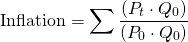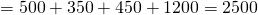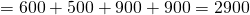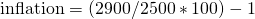# Laspeyres Index

The Laspeyres Index is a kind of price index that is commonly used to measure the level of inflation. The index was first proposed by Étienne Laspeyres in the early 1900s. It was one of the first methods available to calculate inflation and it is still the dominant method today.

On this page we discuss how how to calculate the laspeyres index formula, point out the advantages and disadvantages and how to solve them, and discuss a Laspeyres index formula example.

## Laspeyres price index formula

The Laspeyres price index definition is the following:where Pt is the prices today, P0 is the prices in the previous period, and Q0 is the basket of goods. While the formula looks quite complicated, the intuition is pretty simple. We basically calculate the cost of the basket of goods at current prices, and divided that by the cost of the basket at the old prices. The difference is the amount by which the price level went up, i.e. inflation.

The main advantage of the Laspeyres price index is that it is easy to calculate. Another advantage is that it is a weighted index and thus better captures changes in the prices than an equal-weighted price index.

The main disadvantage of the Laspeyres index is that it is upward biased. This means that it overestimates inflation. There are three reasons why this is the case:

• new goods: older products are replaced by new products. These tend to be more expensive, thereby upward biasing the cost of living (consumer may still be buying the older cheaper substitute good)
• quality changes: if the price of a product increases because the product improved in quality, the price increase should not be considered inflation
• substitution: prices to good relative to each other change and consumers may be substituting goods. This may cause the basket of goods to be unrepresentative of households’ purchases.

To fix the problem with product quality, economists use hedonic pricing. To solve the substitution problem, we can use the Paasche index.

## Laspeyres index vs Paasche index

Next, we discuss the difference between the difference between the Laspeyres and Paasche price index. The Paasche index is an improved version of the Laspeyres index. In particular ,the Paasche index takes into consideration the fact that the basket of goods may have changed. To do this, it updates Q, i.e. the basket of goods.

## Laspeyres index example

Finally, let us consider a simple example: suppose we have 4 goods

• bananas
• train tickets
• gasoline
• watches

Suppose we have a set of prices and quantities, then how do we calculate the index number by the application of laspeyres formula? Suppose we buy 200 bananas, 50 train tickets, 300 gallons of gasoline, and a 100 watches today. The prices for these goods last year were $2.5,$7.00, $1.50,$12.00, respectively. Today, the goods cost $3.00,$10.00, $3, and$9.00.

Applying the formula, we get the index for last yearThe index for this year isInflation equalsor 16%.

## Summary

The Laspeyres is the simplest and most commonly used way to calculate inflation. The approach is very straightforward and can easily be implemented in Excel. A laspeyres index calculator is available for download at the bottom of this page.

### Laspeyres Index calculator Excel

Want to calculate the Paasche index in Excel? Download the Excel file: Laspeyres Index